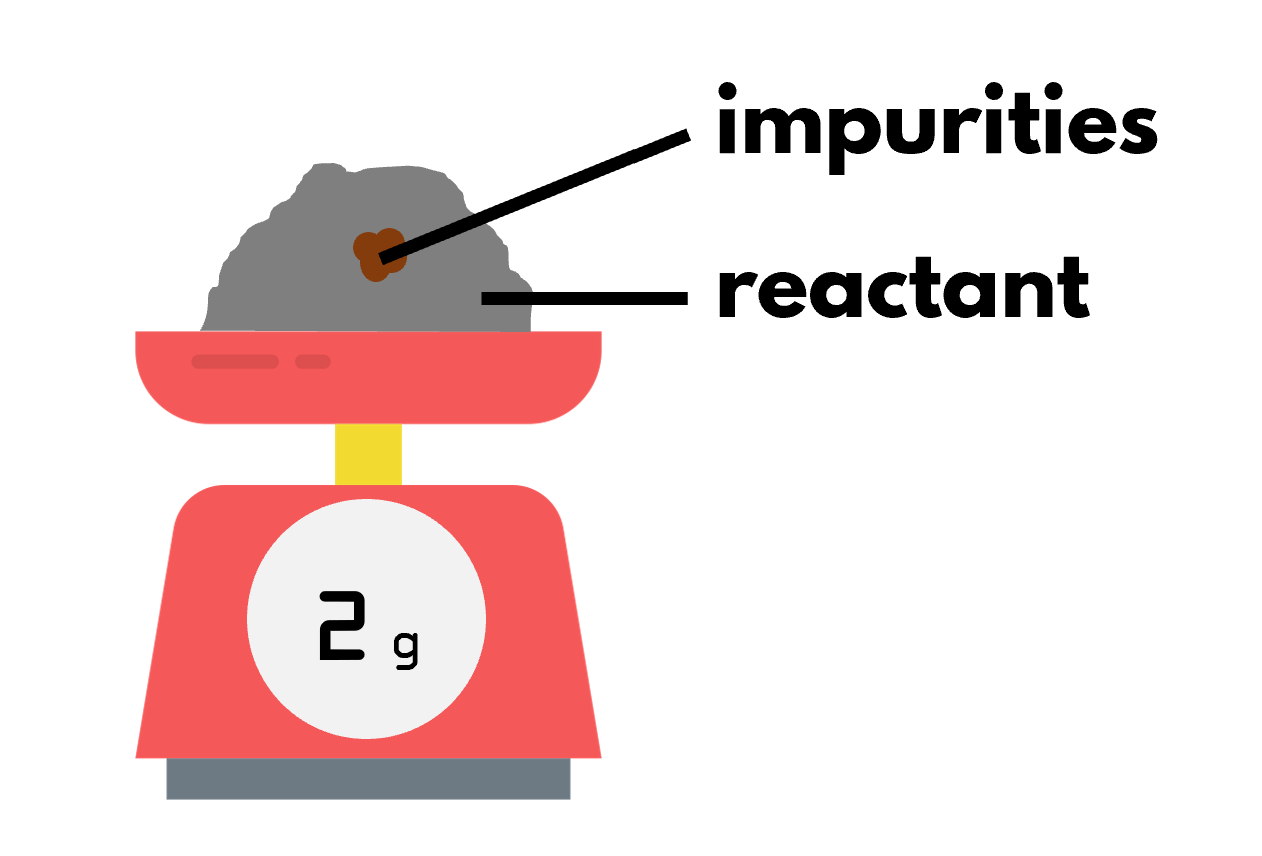CNC Illustration by Gwendolyn Say (@saydrawings)

## 1. The big deal about low yield

Chemistry is a wasteful science. Like how not all the crops in a field will yield fruits, not all the reactants will be converted into products. This gives us a percentage of what we have expected, which in chemistry we call the percentage yield.

A low percentage yield may be due to incomplete reaction, like the reversible reaction to make ammonia. There may also be side reactions to form unwanted side products. Other times, the problem lies with the laboratory technique: we lose some products during the separation steps.

## 2. How to calculate percentage yield

When you look up cheem research papers, the percentage yield of each reaction is written above the arrow. The example above shows a three-step method to synthesise Lacosamide, an important medication for epilepsy. Step 2 is a stumbling block, with the lowest percentage yield of 37%. This means that you only get 37 g of product in the lab, even though you have put in enough reactants to make 100 g of it. To say this in a cheem manner appropriate for the O Level:

• The actual yield of 37 g is the amount of product that is weighed at the end of a reaction
• The theoretical yield of 100 g is the calculated amount of product, assuming that the reaction is 100% efficient
• The percentage yield is the ratio of actual yield to theoretical yield expressed as a percentage: (37 g/100 g) × 100% = 37%

## 3. Formula for percentage yield

percentage yield = actual yield ÷ theoretical yield × 100%

Here, the actual and theoretical yields could be expressed in:

• Number of moles
• Mass (usually for solid products)
• Gaseous volume (usually for gas products)

## 4. Worked example: calculating the percentage yield of salt preparation

In this example, we will be finding the percentage yield of silver iodide formed by precipitation.

10 cm3 of 1 mol/dm3 silver nitrate solution was added to an excess of potassium iodide solution. The silver iodide precipitate was filtered, dried, and weighed. The measured mass was 2 g.

• ### STEP 1: Write the chemical equation

AgNO3 + KI ⟶ AgI + KNO3

• ### STEP 2: Use the amount of limiting reactant to calculate the theoretical yield of product

No. of moles of AgNO3 = concentration × volume in dm3 = 1 × (10/1000) = 0.01 mol

Mole ratio of AgNO3 to AgI = 1:1

No. of moles of AgI = 1/1 × 0.01 = 0.01 mol
Molar mass of AgI = 108 + 127 = 235 g/mol
Theoretical yield of AgI (in mass) = 0.01 × 235 = 2.35 g

• ### STEP 3: Find the percentage mass

Actual yield of AgI = 2 g (given in question)

% yield = (2/2.35) × 100% = 85.1% (3 s.f.)

## 5. Complexity from impurityThe 2 g measured here includes the mass of the impurities.

Another reason why we get less product than expected is due to impurities mixed with reactants. When a sample is impure, its mass we measure includes the mass of impurities. This means that the actual mass of the reactants is lower than expected, translating to less product.

We can quantify how pure a sample is by its percentage purity. It is the percentage of the mass of the pure substance to the mass of the impure sample. In the picture above, a 10% percentage purity would translate to 0.2 g of reactant in 2 g of the impure sample.

## 6. Formula for percentage purity

percentage purity = mass of pure substance ÷ mass of impure sample × 100%

To find the mass of pure substance, we can react a sample and leave the impurities behind. From the amount of product formed, we can back calculate to find out the amount of the pure substance that actually reacted.

## 7. Worked example: calculating the percentage purity of an impure sample of calcium carbonate

In this example, we will calculate the percentage purity of an impure sample containing calcium carbonate. 10 g of the impure sample is heated to decompose any calcium carbonate into calcium oxide and carbon dioxide. Only 2 dm3 of carbon dioxide was formed.

• ### STEP 1: Write the chemical equation

CaCO3 ⟶ CaO + CO2

• ### STEP 2: Work backwards to calculate the mass of pure calcium carbonate

No. of moles of CO2 = gaseous volume ÷ molar volume = 2 ÷ 24 = 0.0833 mol

Mole ratio of CO2 to CaCO3 = 1:1

No. of moles of CaCO3 = (1/1) × 0.0833 = 0.0833 mol
Molar mass of CaCO3 = 40 + 12 + 16×3 = 100 g/mol
Mass of pure CaCO3 = 0.0833 × 100 = 8.3 g

• ### STEP 3: Find the percentage purity

Mass of impure sample = 10 g (given in question)

Percentage purity = (8.33/10) × 100% = 83.3%